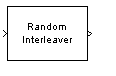Main Content

# Random Interleaver

Reorder input symbols using random permutation

## Library

Block sublibrary of Interleaving

•## Description

The Random Interleaver block rearranges the elements of its input vector using a random permutation. This block accepts a column vector input signal. The Number of elements parameter indicates how many numbers are in the input vector.

The block accepts the following data types: `int8`, `uint8`, `int16`, `uint16`, `int32`, `uint32`, `boolean`, `single`, `double`, and fixed-point. The output signal inherits its data type from the input signal.

The Initial seed parameter initializes the random number generator that the block uses to determine the permutation. The block is predictable for a given seed, but different seeds produce different permutations.

## Parameters

Number of elements

The number of elements in the input vector.

Initial seed

The initial seed value for the random number generator.

## Pair Block

Random Deinterleaver

## See Also

### Blocks

Introduced before R2006a

## Support

#### Bridging Wireless Communications Design and Testing with MATLAB

Download white paper